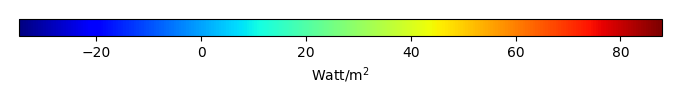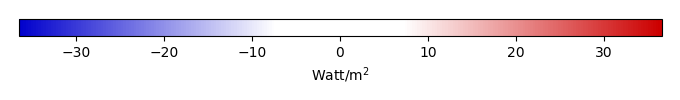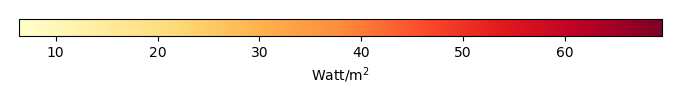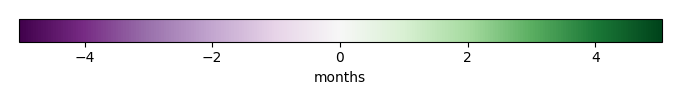# Mean State

Period Mean (original grids) [Watt/m2]
Model Period Mean (intersection) [Watt/m2]
Model Period Mean (complement) [Watt/m2]
Benchmark Period Mean (intersection) [Watt/m2]
Benchmark Period Mean (complement) [Watt/m2]
Bias [Watt/m2]
RMSE [Watt/m2]
Phase Shift [months]
Bias Score 
RMSE Score 
Seasonal Cycle Score 
Spatial Distribution Score 
Overall Score 
Benchmark [-] 44.1
CLM4 [-] 33.4 32.9 44.0 44.2 12.4 -10.8 25.0 1.50 0.259 0.157 0.747 0.786 0.421
CLM4.5 [-] 43.0 42.5 49.2 44.2 12.4 -1.35 17.2 1.43 0.434 0.197 0.764 0.953 0.509
CLM5 [-] 45.4 45.0 52.0 44.2 12.4 1.09 14.3 1.87 0.481 0.266 0.698 0.950 0.532
Period Mean (original grids) [Watt/m2]
Model Period Mean (intersection) [Watt/m2]
Model Period Mean (complement) [Watt/m2]
Benchmark Period Mean (intersection) [Watt/m2]
Benchmark Period Mean (complement) [Watt/m2]
Bias [Watt/m2]
RMSE [Watt/m2]
Phase Shift [months]
Bias Score 
RMSE Score 
Seasonal Cycle Score 
Spatial Distribution Score 
Overall Score 
Benchmark [-] 57.5
CLM4 [-] 45.9 46.1 63.1 57.5 20.8 -11.4 22.5 0.597 0.384 0.388 0.952 0.915 0.605
CLM4.5 [-] 48.4 48.5 66.9 57.5 20.8 -9.01 20.5 0.611 0.426 0.405 0.955 0.856 0.609
CLM5 [-] 50.8 50.9 67.0 57.5 20.8 -6.70 20.0 0.772 0.447 0.430 0.936 0.843 0.617
Period Mean (original grids) [Watt/m2]
Model Period Mean (intersection) [Watt/m2]
Model Period Mean (complement) [Watt/m2]
Benchmark Period Mean (intersection) [Watt/m2]
Benchmark Period Mean (complement) [Watt/m2]
Bias [Watt/m2]
RMSE [Watt/m2]
Phase Shift [months]
Bias Score 
RMSE Score 
Seasonal Cycle Score 
Spatial Distribution Score 
Overall Score 
Benchmark [-] 52.8
CLM4 [-] 50.8 50.7 56.1 53.0 19.6 -2.02 19.8 0.537 0.549 0.420 0.950 0.959 0.659
CLM4.5 [-] 52.3 52.1 57.5 53.0 19.6 -0.550 17.7 0.541 0.570 0.461 0.950 0.975 0.683
CLM5 [-] 56.3 56.1 61.4 53.0 19.6 3.43 17.7 0.747 0.576 0.490 0.915 0.971 0.688
Period Mean (original grids) [Watt/m2]
Model Period Mean (intersection) [Watt/m2]
Model Period Mean (complement) [Watt/m2]
Benchmark Period Mean (intersection) [Watt/m2]
Benchmark Period Mean (complement) [Watt/m2]
Bias [Watt/m2]
RMSE [Watt/m2]
Phase Shift [months]
Bias Score 
RMSE Score 
Seasonal Cycle Score 
Spatial Distribution Score 
Overall Score 
Benchmark [-] 18.0
CLM4 [-] 15.7 16.1 8.52 18.1 5.64 -2.47 21.6 0.527 0.792 0.455 0.963 0.954 0.724
CLM4.5 [-] 15.6 16.0 8.75 18.1 5.64 -2.58 18.6 0.528 0.801 0.518 0.964 0.963 0.753
CLM5 [-] 15.6 16.0 7.19 18.1 5.64 -2.63 19.1 0.548 0.786 0.515 0.959 0.930 0.741
Period Mean (original grids) [Watt/m2]
Model Period Mean (intersection) [Watt/m2]
Model Period Mean (complement) [Watt/m2]
Benchmark Period Mean (intersection) [Watt/m2]
Benchmark Period Mean (complement) [Watt/m2]
Bias [Watt/m2]
RMSE [Watt/m2]
Phase Shift [months]
Bias Score 
RMSE Score 
Seasonal Cycle Score 
Spatial Distribution Score 
Overall Score 
Benchmark [-] 22.1
CLM4 [-] 2.67 0.0105 16.0 22.8 10.8 -18.5 27.9 1.60 0.0685 0.122 0.756 0.356 0.285
CLM4.5 [-] 24.0 22.4 31.7 22.8 10.8 2.21 16.8 1.36 0.261 0.159 0.800 0.784 0.433
CLM5 [-] 32.3 31.3 36.9 22.8 10.8 10.5 16.4 1.34 0.305 0.199 0.799 0.866 0.474
Period Mean (original grids) [Watt/m2]
Model Period Mean (intersection) [Watt/m2]
Model Period Mean (complement) [Watt/m2]
Benchmark Period Mean (intersection) [Watt/m2]
Benchmark Period Mean (complement) [Watt/m2]
Bias [Watt/m2]
RMSE [Watt/m2]
Phase Shift [months]
Bias Score 
RMSE Score 
Seasonal Cycle Score 
Spatial Distribution Score 
Overall Score 
Benchmark [-] 27.0
CLM4 [-] 27.6 27.7 24.5 27.1 8.81 0.785 12.7 0.371 0.823 0.650 0.972 0.926 0.804
CLM4.5 [-] 21.8 21.9 25.1 27.1 8.81 -5.08 14.7 0.686 0.755 0.615 0.935 0.974 0.779
CLM5 [-] 20.6 20.5 27.0 27.1 8.81 -6.35 15.9 0.553 0.733 0.601 0.949 0.935 0.764
Period Mean (original grids) [Watt/m2]
Model Period Mean (intersection) [Watt/m2]
Model Period Mean (complement) [Watt/m2]
Benchmark Period Mean (intersection) [Watt/m2]
Benchmark Period Mean (complement) [Watt/m2]
Bias [Watt/m2]
RMSE [Watt/m2]
Phase Shift [months]
Bias Score 
RMSE Score 
Seasonal Cycle Score 
Spatial Distribution Score 
Overall Score 
Benchmark [-] 28.4
CLM4 [-] 34.0 33.8 37.9 28.4 24.4 5.32 19.5 1.01 0.642 0.495 0.876 0.934 0.689
CLM4.5 [-] 32.7 32.4 38.1 28.4 24.4 3.93 19.2 0.921 0.655 0.495 0.888 0.933 0.693
CLM5 [-] 35.5 35.2 39.6 28.4 24.4 6.80 19.3 0.795 0.664 0.515 0.920 0.937 0.710
Period Mean (original grids) [Watt/m2]
Model Period Mean (intersection) [Watt/m2]
Model Period Mean (complement) [Watt/m2]
Benchmark Period Mean (intersection) [Watt/m2]
Benchmark Period Mean (complement) [Watt/m2]
Bias [Watt/m2]
RMSE [Watt/m2]
Phase Shift [months]
Bias Score 
RMSE Score 
Seasonal Cycle Score 
Spatial Distribution Score 
Overall Score 
Benchmark [-] 40.3
CLM4 [-] 33.3 33.1 35.7 40.6 11.0 -7.99 21.7 0.551 0.467 0.440 0.957 0.941 0.649
CLM4.5 [-] 37.9 37.7 41.3 40.6 11.0 -3.11 19.7 0.534 0.536 0.467 0.959 0.838 0.653
CLM5 [-] 37.6 37.1 42.1 40.6 11.0 -3.33 20.5 0.882 0.499 0.461 0.910 0.879 0.642
Period Mean (original grids) [Watt/m2]
Model Period Mean (intersection) [Watt/m2]
Model Period Mean (complement) [Watt/m2]
Benchmark Period Mean (intersection) [Watt/m2]
Benchmark Period Mean (complement) [Watt/m2]
Bias [Watt/m2]
RMSE [Watt/m2]
Phase Shift [months]
Bias Score 
RMSE Score 
Seasonal Cycle Score 
Spatial Distribution Score 
Overall Score 
Benchmark [-] 15.2
CLM4 [-] 9.70 10.2 2.25 15.2 3.78 -5.26 21.1 0.793 0.790 0.467 0.945 0.954 0.725
CLM4.5 [-] 6.99 7.38 0.646 15.2 3.78 -8.07 20.0 0.724 0.705 0.504 0.945 0.910 0.713
CLM5 [-] 4.37 4.77 -2.26 15.2 3.78 -10.7 22.1 0.816 0.642 0.486 0.933 0.847 0.679
Period Mean (original grids) [Watt/m2]
Model Period Mean (intersection) [Watt/m2]
Model Period Mean (complement) [Watt/m2]
Benchmark Period Mean (intersection) [Watt/m2]
Benchmark Period Mean (complement) [Watt/m2]
Bias [Watt/m2]
RMSE [Watt/m2]
Phase Shift [months]
Bias Score 
RMSE Score 
Seasonal Cycle Score 
Spatial Distribution Score 
Overall Score 
Benchmark [-] 56.5
CLM4 [-] 58.3 58.7 49.3 56.9 15.0 1.34 17.3 0.703 0.696 0.559 0.949 0.885 0.730
CLM4.5 [-] 58.4 58.7 52.3 56.9 15.0 1.47 17.9 0.697 0.689 0.551 0.947 0.813 0.710
CLM5 [-] 59.7 60.1 51.0 56.9 15.0 2.71 15.4 0.540 0.712 0.620 0.962 0.893 0.761
Period Mean (original grids) [Watt/m2]
Model Period Mean (intersection) [Watt/m2]
Model Period Mean (complement) [Watt/m2]
Benchmark Period Mean (intersection) [Watt/m2]
Benchmark Period Mean (complement) [Watt/m2]
Bias [Watt/m2]
RMSE [Watt/m2]
Phase Shift [months]
Bias Score 
RMSE Score 
Seasonal Cycle Score 
Spatial Distribution Score 
Overall Score 
Benchmark [-] 20.2
CLM4 [-] 21.2 20.8 29.3 20.4 7.08 1.27 16.0 0.935 0.757 0.575 0.903 0.874 0.736
CLM4.5 [-] 20.4 20.0 28.9 20.4 7.08 0.509 15.7 0.859 0.739 0.596 0.913 0.832 0.735
CLM5 [-] 19.6 19.2 29.0 20.4 7.08 -0.160 16.0 0.832 0.722 0.598 0.916 0.822 0.731
Period Mean (original grids) [Watt/m2]
Model Period Mean (intersection) [Watt/m2]
Model Period Mean (complement) [Watt/m2]
Benchmark Period Mean (intersection) [Watt/m2]
Benchmark Period Mean (complement) [Watt/m2]
Bias [Watt/m2]
RMSE [Watt/m2]
Phase Shift [months]
Bias Score 
RMSE Score 
Seasonal Cycle Score 
Spatial Distribution Score 
Overall Score 
Benchmark [-] 34.3
CLM4 [-] 28.7 33.7 8.31 34.6 11.5 -1.01 21.1 0.849 0.556 0.450 0.915 0.926 0.660
CLM4.5 [-] 30.0 35.1 9.33 34.6 11.5 0.459 19.4 0.815 0.592 0.463 0.922 0.937 0.675
CLM5 [-] 31.0 36.8 6.87 34.6 11.5 2.16 19.1 0.881 0.599 0.490 0.911 0.925 0.683
Period Mean (original grids) [Watt/m2]
Model Period Mean (intersection) [Watt/m2]
Model Period Mean (complement) [Watt/m2]
Benchmark Period Mean (intersection) [Watt/m2]
Benchmark Period Mean (complement) [Watt/m2]
Bias [Watt/m2]
RMSE [Watt/m2]
Phase Shift [months]
Bias Score 
RMSE Score 
Seasonal Cycle Score 
Spatial Distribution Score 
Overall Score 
Benchmark [-] 22.7
CLM4 [-] 45.1 46.8 43.5 22.8 18.3 24.7 36.1 1.09 0.505 0.500 0.948 0.765 0.644
CLM4.5 [-] 45.2 47.1 43.4 22.8 18.3 25.1 36.2 1.09 0.505 0.499 0.948 0.773 0.645
CLM5 [-] 45.5 48.2 43.0 22.8 18.3 26.2 36.6 1.26 0.509 0.485 0.937 0.776 0.639
Period Mean (original grids) [Watt/m2]
Model Period Mean (intersection) [Watt/m2]
Model Period Mean (complement) [Watt/m2]
Benchmark Period Mean (intersection) [Watt/m2]
Benchmark Period Mean (complement) [Watt/m2]
Bias [Watt/m2]
RMSE [Watt/m2]
Phase Shift [months]
Bias Score 
RMSE Score 
Seasonal Cycle Score 
Spatial Distribution Score 
Overall Score 
Benchmark [-] 17.0
CLM4 [-] 13.1 13.9 2.15 17.0 4.51 -3.84 20.0 0.610 0.780 0.522 0.962 0.870 0.731
CLM4.5 [-] 10.6 11.1 2.08 17.0 4.51 -6.38 18.4 0.690 0.737 0.555 0.952 0.914 0.743
CLM5 [-] 9.34 9.87 0.733 17.0 4.51 -7.63 21.0 0.966 0.705 0.511 0.907 0.884 0.704
Period Mean (original grids) [Watt/m2]
Model Period Mean (intersection) [Watt/m2]
Model Period Mean (complement) [Watt/m2]
Benchmark Period Mean (intersection) [Watt/m2]
Benchmark Period Mean (complement) [Watt/m2]
Bias [Watt/m2]
RMSE [Watt/m2]
Phase Shift [months]
Bias Score 
RMSE Score 
Seasonal Cycle Score 
Spatial Distribution Score 
Overall Score 
Benchmark [-] 47.7
CLM4 [-] 46.5 46.2 51.6 48.2 15.9 -2.29 22.5 0.615 0.514 0.488 0.942 0.907 0.668
CLM4.5 [-] 47.0 46.5 54.2 48.2 15.9 -1.70 23.7 0.576 0.495 0.477 0.945 0.904 0.660
CLM5 [-] 50.2 49.8 55.9 48.2 15.9 1.46 21.3 0.676 0.515 0.534 0.932 0.885 0.680
Period Mean (original grids) [Watt/m2]
Model Period Mean (intersection) [Watt/m2]
Model Period Mean (complement) [Watt/m2]
Benchmark Period Mean (intersection) [Watt/m2]
Benchmark Period Mean (complement) [Watt/m2]
Bias [Watt/m2]
RMSE [Watt/m2]
Phase Shift [months]
Bias Score 
RMSE Score 
Seasonal Cycle Score 
Spatial Distribution Score 
Overall Score 
Benchmark [-] 28.6
CLM4 [-] 23.8 23.7 22.9 28.7 12.1 -4.86 16.7 0.927 0.632 0.400 0.910 0.960 0.660
CLM4.5 [-] 23.5 23.4 25.5 28.7 12.1 -5.26 15.7 0.767 0.634 0.430 0.923 0.877 0.659
CLM5 [-] 30.5 30.4 32.8 28.7 12.1 1.84 14.4 0.637 0.727 0.471 0.933 0.981 0.717
Period Mean (original grids) [Watt/m2]
Model Period Mean (intersection) [Watt/m2]
Model Period Mean (complement) [Watt/m2]
Benchmark Period Mean (intersection) [Watt/m2]
Benchmark Period Mean (complement) [Watt/m2]
Bias [Watt/m2]
RMSE [Watt/m2]
Phase Shift [months]
Bias Score 
RMSE Score 
Seasonal Cycle Score 
Spatial Distribution Score 
Overall Score 
Benchmark [-] 26.7
CLM4 [-] 31.1 31.2 20.6 26.9 8.98 4.07 13.8 0.396 0.789 0.663 0.970 0.962 0.810
CLM4.5 [-] 25.5 25.6 22.4 26.9 8.98 -1.44 14.2 0.697 0.818 0.628 0.932 0.959 0.793
CLM5 [-] 24.9 24.9 24.5 26.9 8.98 -1.95 16.2 0.693 0.785 0.593 0.937 0.969 0.775
Period Mean (original grids) [Watt/m2]
Model Period Mean (intersection) [Watt/m2]
Model Period Mean (complement) [Watt/m2]
Benchmark Period Mean (intersection) [Watt/m2]
Benchmark Period Mean (complement) [Watt/m2]
Bias [Watt/m2]
RMSE [Watt/m2]
Phase Shift [months]
Bias Score 
RMSE Score 
Seasonal Cycle Score 
Spatial Distribution Score 
Overall Score 
Benchmark [-] 49.7
CLM4 [-] 42.7 42.4 65.8 49.8 11.0 -7.44 17.8 0.621 0.674 0.657 0.942 0.889 0.764
CLM4.5 [-] 41.0 40.6 69.9 49.8 11.0 -9.12 19.5 0.602 0.637 0.642 0.942 0.926 0.758
CLM5 [-] 44.9 44.6 66.6 49.8 11.0 -5.13 17.0 0.824 0.726 0.646 0.923 0.891 0.766
Period Mean (original grids) [Watt/m2]
Model Period Mean (intersection) [Watt/m2]
Model Period Mean (complement) [Watt/m2]
Benchmark Period Mean (intersection) [Watt/m2]
Benchmark Period Mean (complement) [Watt/m2]
Bias [Watt/m2]
RMSE [Watt/m2]
Phase Shift [months]
Bias Score 
RMSE Score 
Seasonal Cycle Score 
Spatial Distribution Score 
Overall Score 
Benchmark [-] 30.2
CLM4 [-] 12.2 12.0 38.3 30.3 13.0 -17.4 26.8 1.65 0.139 0.141 0.782 0.363 0.313
CLM4.5 [-] 29.0 28.8 44.7 30.3 13.0 -0.872 15.8 1.22 0.435 0.193 0.854 0.678 0.471
CLM5 [-] 30.7 30.7 45.7 30.3 13.0 0.943 12.6 1.42 0.427 0.277 0.810 0.701 0.498
Period Mean (original grids) [Watt/m2]
Model Period Mean (intersection) [Watt/m2]
Model Period Mean (complement) [Watt/m2]
Benchmark Period Mean (intersection) [Watt/m2]
Benchmark Period Mean (complement) [Watt/m2]
Bias [Watt/m2]
RMSE [Watt/m2]
Phase Shift [months]
Bias Score 
RMSE Score 
Seasonal Cycle Score 
Spatial Distribution Score 
Overall Score 
Benchmark [-] 40.3
CLM4 [-] 36.8 37.0 35.6 40.4 9.36 -3.39 18.0 1.06 0.549 0.341 0.889 0.815 0.587
CLM4.5 [-] 40.1 40.3 38.1 40.4 9.36 -0.0546 15.9 0.881 0.634 0.360 0.916 0.916 0.637
CLM5 [-] 42.8 43.1 40.0 40.4 9.36 2.70 14.4 0.875 0.607 0.430 0.911 0.875 0.651
Period Mean (original grids) [Watt/m2]
Model Period Mean (intersection) [Watt/m2]
Model Period Mean (complement) [Watt/m2]
Benchmark Period Mean (intersection) [Watt/m2]
Benchmark Period Mean (complement) [Watt/m2]
Bias [Watt/m2]
RMSE [Watt/m2]
Phase Shift [months]
Bias Score 
RMSE Score 
Seasonal Cycle Score 
Spatial Distribution Score 
Overall Score 
Benchmark [-] 41.5
CLM4 [-] 59.6 58.7 68.1 42.0 6.56 15.4 33.9 0.801 0.518 0.483 0.929 0.829 0.649
CLM4.5 [-] 59.5 58.8 68.4 42.0 6.56 15.7 33.5 0.709 0.518 0.490 0.944 0.848 0.658
CLM5 [-] 62.7 62.0 68.8 42.0 6.56 18.5 33.8 0.746 0.500 0.512 0.939 0.830 0.659

# Temporally integrated period mean

BENCHMARK MEANMODEL MEANBIASBIAS SCORERMSERMSE SCOREBENCHMARK MAX MONTHMODEL MAX MONTHDIFFERENCE IN MAX MONTHSEASONAL CYCLE SCORESPATIAL TAYLOR DIAGRAMMODEL COLORS# Spatially integrated regional mean

MODEL COLORSREGIONAL MEANANNUAL CYCLEMONTHLY ANOMALYANNUAL CYCLE# All Models

BenchmarkCLM4CLM4.5CLM5# Data Information

creation_date: Tue Jul 28 15:54:11 PDT 2015

source_file: This product is generated from monthly Global Biosphere Amtospher Flux (GBAF) observations

title: derived GBAF in 0.5x0.5

Approach: I read this variable from data file, and then save the data in NetCDF format by each month and each year. Meanwhile, I also converted the unit from MJoule*m-2*d-1 to Watt/m2.

Temporal resolution: monthly

General information: This product was derived from Monthly estimates of global biosphere-atmosphere fluxes from Biogeochemistry group at Max Planck Institute in Jena, Germany.

Spatial resolution: 0.5x0.5 degree